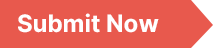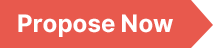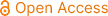### International Journal of Systems Science and Applied Mathematics

Archive
• 2023, Volume 8

• 2022, Volume 7

• 2021, Volume 6

• 2020, Volume 5

• 2019, Volume 4

• 2018, Volume 3

• 2017, Volume 2

• 2016, Volume 1

Submit a Manuscript

Publishing with us to make your research visible to the widest possible audience.Propose a Special Issue

Building a community of authors and readers to discuss the latest research and develop new ideas.### An Analysis of Solutions of Nonlinear Equations Using AI Inspired Mathematical Packages

In the era of Artificial Intelligence (AI), achieving precise solutions for nonlinear equations has been considerably streamlined, thanks to the advancement of various mathematical tools designed for numerical computations. However, as the utilization of these mathematical software continues to rise, researchers are keen to ascertain the optimal choice among these tools based on their outcome when applied to solving nonlinear equations. This study addresses this question by undertaking a comparative analysis of three prominent mathematical software packages Python, Scilab, and MATLAB using two numerical approaches: Newton-Raphson and Secant. By employing the Newton-Raphson and Secant methods to solve five benchmark problems, this paper assesses the performance of the aforementioned mathematical tools. Notably, the outcomes underscore the competence of all three software options in yielding suitable approximations of the problem's root solutions. In particular, Python stands out for its ability to achieve this while utilizing the fewest iterations and minimizing computational time. As a result, among the three tools investigated, Python emerges as the most favorable choice, considering its efficiency and accuracy. Furthermore, this research validates the robustness of the Newton-Raphson approach over the Secant method, given its capability to efficiently converge to the solutions with the minimal iteration count across the benchmark problems. This finding highlights the superiority of the Newton-Raphson method as a more efficient and reliable technique for solving the considered benchmark problems.

Nonlinear Equations, Artificial Intelligence (AI), MATLAB, SCILAB, Python, Secant Method, Newton Raphson Method

Isaac Azure. (2023). An Analysis of Solutions of Nonlinear Equations Using AI Inspired Mathematical Packages. International Journal of Systems Science and Applied Mathematics, 8(2), 23-30. https://doi.org/10.11648/j.ijssam.20230802.12